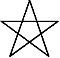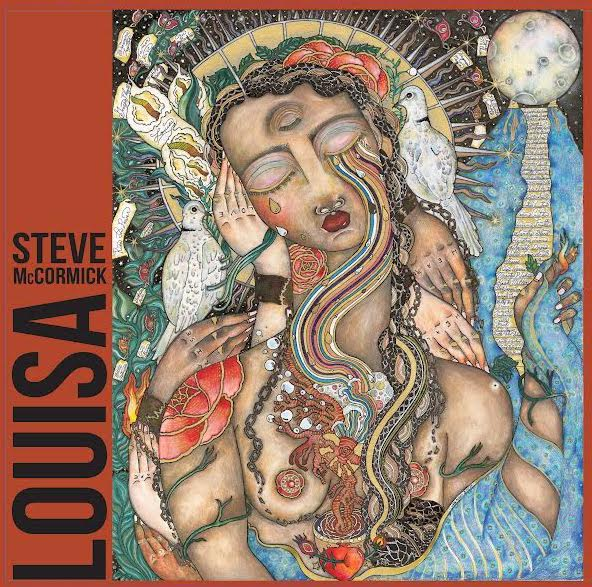Art, History, Music, Politics, and Spirituality For The Modern Alchemist – circulation in over 129 countries

# The Sign of Shamhat Year 18,003 Begins May 9th 2015

There are eight months in the Calendar of Mu, sometimes referred to as the Nyarzirian Calendar. Each month is consists of forty-five days, and represents a system of the eight hexagrams moving through the five elements. The Ninzuwu Shaman invokes the mudra/mantra formula associated with each hexagram that rules a particular day after invoking the Armor of Amaterasu Ohkami. The chart below illustrates the daily Vasuh letters invoked and the ruling “Ninzuwu” for the said hexagram:
1. May 9th 2015 = Aozu = 17thHexagram = Phe-Hmu-Tuu
2. May 10th2015 = Asakhira = 28thHexagram = Aum-Nzu-Bnhu
3. May 11th2015 = Zwa = 31st Hexagram = Aum-Aum-Hmu
4. May 12th2015 = Chuki = 43rdHexagram = Zhee-Zhee-Phe
5. May 13th2015 = Lalui = 45thHexagram = Nzu-Hmu-Lewhu-Tuu-Nzu
6. May 14th2015 = Nhu = 47thHexagram = Shki-Zhee-Bnhu
7. May 15th2015 = Butz = 49thHexagram = Hmu-Shki-Aum
8. May 16th2015 = Shamhat = 58thHexagram = Bnhu-Aum
9. May 17th2015 =Entering Earth Element
10. May 18th2015 = Aozu = 17thHexagram = Phe-Hmu-Tuu
11. May 19th2015 = Asakhira = 28thHexagram = Aum-Nzu-Bnhu
12. May 20th 2015 = Zwa = 31st Hexagram = Aum-Aum-Hmu
13. May 21st2015 = Chuki = 43rdHexagram = Zhee-Zhee-Phe
14. May 22nd2015 = Lalui = 45thHexagram = Nzu-Hmu-Lewhu-Tuu-Nzu
15. May 23rd2015 = Nhu = 47thHexagram = Shki-Zhee-Bnhu
16. May 24th2015 = Butz = 49thHexagram = Hmu-Shki-Aum
17. May 25th2015 = Shamhat = 58thHexagram = Bnhu-Aum
18. May 26th 2015 =Entering Water Element
19. May 27th2015 = Aozu = 17thHexagram = Phe-Hmu-Tuu
20. May 28th 2015 = Asakhira = 28thHexagram = Aum-Nzu-Bnhu
21. May 29th 2015 = Zwa = 31st Hexagram = Aum-Aum-Hmu
22. May 30th 2015 = Chuki = 43rdHexagram = Zhee-Zhee-Phe
23. May 31st 2015 = Lalui = 45thHexagram = Nzu-Hmu-Lewhu-Tuu-Nzu
24. June 1st2015 = Nhu = 47thHexagram = Shki-Zhee-Bnhu
25. June 2nd2015 = Butz = 49thHexagram = Hmu-Shki-Aum
26. June 3rd2015 = Shamhat = 58thHexagram = Bnhu-Aum
27. June 4th2015 =Entering Fire Element
28. June 5th2015 = Aozu = 17thHexagram = Phe-Hmu-Tuu
29. June 6th2015 = Asakhira = 28thHexagram = Aum-Nzu-Bnhu
30. June 7th2015 = Zwa = 31st Hexagram = Aum-Aum-Hmu
31. June 8th2015 = Chuki = 43rdHexagram = Zhee-Zhee-Phe
32. June 9th2015 = Lalui = 45thHexagram = Nzu-Hmu-Lewhu-Tuu-Nzu
33. June 10th2015 = Nhu = 47thHexagram = Shki-Zhee-Bnhu
34. June 11th2015 = Butz = 49thHexagram = Hmu-Shki-Aum
35. June 12th2015 = Shamhat = 58thHexagram = Bnhu-Aum
36. June 13th2015 =Entering Air Element
37. June 14th2015 = Aozu = 17thHexagram = Phe-Hmu-Tuu
38. June 15th2015 = Asakhira = 28thHexagram = Aum-Nzu-Bnhu
39. June 16th2015 = Zwa = 31st Hexagram = Aum-Aum-Hmu
40. June 17th2015 = Chuki = 43rdHexagram = Zhee-Zhee-Phe
41. June 18th2015 = Lalui = 45thHexagram = Nzu-Hmu-Lewhu-Tuu-Nzu
42. June 19th2015 = Nhu = 47thHexagram = Shki-Zhee-Bnhu
43. June 20th2015 = Butz = 49thHexagram = Hmu-Shki-Aum
44. June 21st2015 = Shamhat = 58thHexagram = Bnhu-Aum
45. June 22nd2015

#### You may have missed#### Louisa – Steve McCormick#### Bootlegger Blood – speakeazie